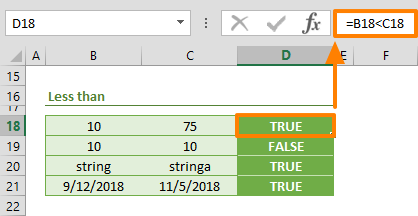Excel comparison operators can compare two values and return a logical (Boolean) value. You can use the return logical value in other functions like IF, or features, like Conditional Formatting, that require a logical value input. In this guide, we are going to show you various types of Excel comparison operators available, and go over some tips and examples. Let's start with a few tips:

• A logical comparison always returns a Boolean value. Per its definition, this means that there are two possible outcomes (Boolean): TRUE and FALSE.
• The comparisons are formulas. You do not need any other functions to execute them. Simply begin with an equal operator (=) like starting a regular formula:

=10=10 returns TRUE

=10=75 returns FALSE

• You can use these operators everywhere a logical test is allowed. For example, you can use these operators in IF, AND, OR and NOT functions:

=IF(A1>A2,"Passed","Failed")

=IF(AND(A5=G1,MyValue<=10),B5+1,B5-1)

• We recommend using the EXACT function to compare strings.

# Excel Comparison Operators

## Equal to

The equal to operator (=) returns TRUE if two values are equal to each other, otherwise FALSE.## Greater than

The greater than operator (>) returns TRUE if the first value is greater than the second value, and returns FALSE otherwise.## Less than

The less than operator (<) returns TRUE if the first value is less than the second value, and returns FALSE otherwise.## Greater than or equal to

The greater than or equal to operator (>=) returns TRUE if the first value is greater than or equal to the second value, and returns FALSE otherwise.## Less than or equal to

The less than or equal to operator (<=) returns TRUE if the first value is less than or equal to the second value, and returns FALSE otherwise.## Not Equal to

The not equal to operator (<>) returns TRUE if two values are not equal to each other, and returns FALSE otherwise.Alternatively, you can use the NOT function with the equal to operator to essentially run the same test.